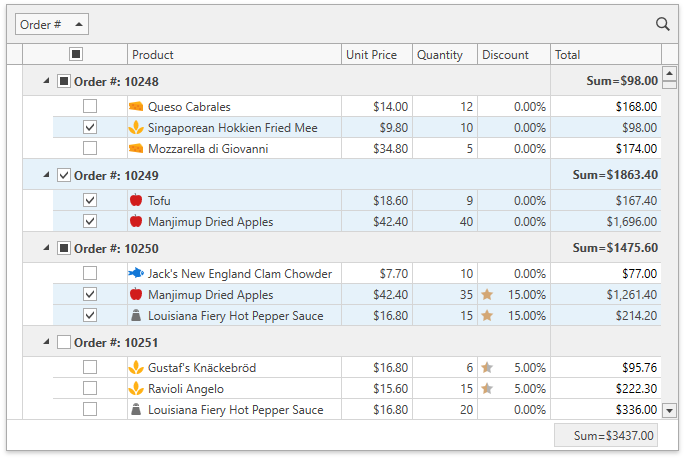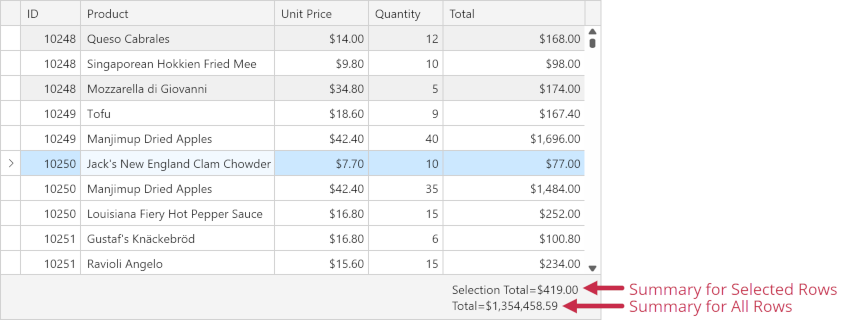# DataViewBase.SummaryCalculationMode Property

Gets or sets a mode that specifies by which rows the summary value is calculated.

Namespace: DevExpress.Xpf.Grid

Assembly: DevExpress.Xpf.Grid.v22.2.Core.dll

NuGet Package: DevExpress.Wpf.Grid.Core

## Declaration

``public GridSummaryCalculationMode SummaryCalculationMode { get; set; }``

## Property Value

Type Description
GridSummaryCalculationMode

A mode that specifies by which rows the summary value is calculated.

Available values:

Name Description
AllRows

The summary value is calculated against all rows.

SelectedRows

The summary value is calculated against selected rows.

Mixed

The summary value is calculated against selected rows if their number is more than one; otherwise, against all rows.

## Remarks

The GridControl can calculate summaries against selected rows and cells:Set the DataViewBase.SummaryCalculationMode property to SelectedRows / Mixed to make the GridControl calculate all summaries within its view against selected rows:

``````<dxg:GridControl x:Name="grid" SelectionMode="Row">
<dxg:GridControl.View>
<dxg:TableView x:Name="view"
SummaryCalculationMode="SelectedRows"
TotalSummaryPosition="Bottom" />
</dxg:GridControl.View>
<dxg:GridControl.TotalSummary>
<dxg:GridSummaryItem FieldName="Total" SummaryType="Sum" DisplayFormat="Sum={0:\$0.00}" />
</dxg:GridControl.TotalSummary>
<dxg:GridControl.GroupSummary>
<dxg:GridSummaryItem FieldName="Total" SummaryType="Sum" DisplayFormat="Sum={0:\$0.00}" />
</dxg:GridControl.GroupSummary>
</dxg:GridControl>
``````

You can create an individual summary for selected rows/cells and set its SummaryItemBase.CalculationMode property to SelectedRows / Mixed:``````<dxg:GridControl x:Name="grid" SelectionMode="Row">
<!-- -->
<dxg:GridControl.View>
<dxg:TableView x:Name="view" TotalSummaryPosition="Bottom" ShowGroupedColumns="True" />
</dxg:GridControl.View>
<dxg:GridControl.TotalSummary>
<!-- The first summary against selected rows -->
<dxg:GridSummaryItem FieldName="Total" SummaryType="Sum" ShowInColumn="Total"
DisplayFormat="Selection Total=\${0:N}" CalculationMode="SelectedRows" />
<!-- The second summary against all rows -->
<dxg:GridSummaryItem FieldName="Total" SummaryType="Sum" ShowInColumn="Total"
DisplayFormat="Total=\${0:N}" />
</dxg:GridControl.TotalSummary>
<dxg:GridControl.GroupSummary>
<dxg:GridSummaryItem FieldName="Total" SummaryType="Sum"
DisplayFormat="Grand Total=\${0:N}" CalculationMode="SelectedRows" />
</dxg:GridControl.GroupSummary>
</dxg:GridControl>
``````

Note

The GridControl in Server Mode or bound to ICollectionView does not calculate summaries for selection.